×
Get Full Access to UGA - CHEM 1212 - Class Notes - Week 6
Get Full Access to UGA - CHEM 1212 - Class Notes - Week 6

×

UGA / Chemistry / CHEM 1212 / What do you call the study of the rates and mechanisms of chemical rea

# What do you call the study of the rates and mechanisms of chemical rea Description

##### Description: class notes with detailed textbook notes
8 Pages 195 Views 1 Unlocks
Reviews

CHAPTER 14

## What do you call the study of the rates and mechanisms of chemical reactions?Factors that Influence Reactivity

∙ Chemical kinetics

o the study of reaction mechanisms and the rates of chemical reactions  under various conditions

∙ For a reaction to occur to an appreciable amount, it must be both  thermodynamically and kinetically favored

o Thermodynamic controls

 the enthalpy change for the reaction

 the entropy change for the reaction

 the temperature at which the reaction takes place

o kinetic controls

 the manner in which the reaction takes place (its mechanism)  the energy barrier that must be overcome to convert reactants  to products (the activation energy) We also discuss several other topics like How to innovate?

 the concentration of the species present

## What is kinetic control in a reaction? the temperature at which the reaction takes place

Collision Theory

∙ conditions

o The reacting species come into contact (they collide)

 When more collisions between reacting species occur, the

reaction will proceed faster We also discuss several other topics like What is the content of the code of hammurabi – 1754 bce?

o The collision has enough energy to overcome the activation energy,  the energy barrier necessary to initiate the reaction

 only some fraction of reacting species will have enough energy  to react at a given temperature

 As the temperature increases, the number of species with

enough energy to react will increase

∙ the high-temperature sample will have more energetic

collisions and will experience a faster reaction rate

## How do you calculate average rate of reaction?We also discuss several other topics like What is the definition of integration by parts?

 a greater fraction of reactant molecules have enough energy to  overcome a small activation energy than to overcome a large

activation energy

o The reacting species collide in an orientation that allows the necessary  bond breaking and bond forming needed to transform reactants to  products to take place

Average Rate vs Reaction Stoichiometry

∙ Reaction rate

o the speed at which a reaction progresses, as a ratio of change in  concentration over change in time

o Because reactants decrease over time, the change in its concentration  is negative

∙ Average Reaction Rate

o the change in concentration of a reactant or product over a defined  time interval

∙ Stoichiometry

o relative rate at which reactants are consumed and products are formed is directly related to the reaction stoichiometry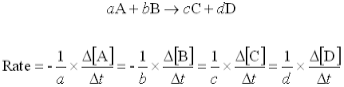Instantaneous and Initial Rates

∙ instantaneous rate

o the rate of a reaction at that point in time, is equal to the slope of a  line tangent to the concentration–time curve at a given point in time ∙ Initial Rate Don't forget about the age old question of What is the quality of sound that distinguishes different voices and instruments from each other called?

o the rate of the reaction at the beginning of the reaction, when t=0 o start of chemical reaction

∙ change over time

o concentration versus change is plotted on a graph to see the  disappearance of reactant related to the creation of product

Concentration and Reaction Rate

∙ rate law

o shows the quantitative relationship between reaction rate and the  concentration of species involved in the chemical reaction

∙ catalyst

o a species that speeds up the rate of a chemical reaction but does not  undergo any permanent chemical change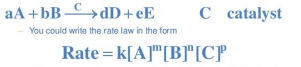∙ components of rate laws

o rate constant, k

 the proportionality constant in the rate equation Don't forget about the age old question of What is plato's theory of ideal forms?

 value of k, which is determined from experiments, is unique to a given reaction and varies with temperature

o Concentrations of reacting species (in this example A, B, and the catalyst, C)

 includes the concentrations of reacting species in moles per liter (for species in solution) or pressure units (for gases)

 Not all species that participate in a reaction will always appear in the rate law, and, as is true for catalysts, it is possible for a species that does not appear in the overall reaction to appear in the rate law

o The order of the reaction with respect to the reacting species (in this  example, m, n, and p)

 Reaction order

∙ expresses the order of the reaction with respect to each

reactant, and it shows the effect a reacting species has on

the rate of the reaction Don't forget about the age old question of How much is a mole?

 Reaction orders are determined from experiments; they are not taken from the stoichiometric coefficients in the balanced equation

 Overall reaction order

∙ Sum of the individual reaction orders

Determining Rate Law using Initial Rates

∙ Method of initial rates

o a reaction under investigation is repeated multiple times, and each experiment has a different set of initial concentrations for the reactants o initial rate is measured for each experiment, and the results are used to determine the order of the reaction with respect to each reacting species

o the concentration of only one reacting species is changed between sets of experiments while the other concentrations do not change

rate2 = (concentration in 2nd

experiment)z

rate1 (concentration in 1st experiment)

 the entire right side is raised to the power of z

 z=reaction order

Integrated Rate Laws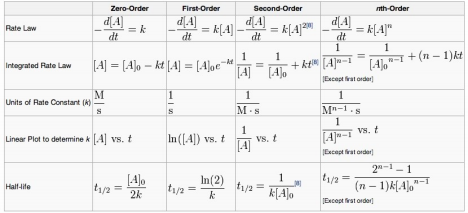∙ derived by integration of a rate law equation

Graphical Determination of Reaction Order

∙ Integrated rate laws for zero-, first-, and second-order reactions can be  rewritten in the form of an equation for a straight line, y=mx+b ∙ Steps

o Collect concentration–time data for a reaction at some temperature. o Plot the concentration–time data three different ways:

 Concentration versus time

 Natural log of concentration versus time

 1/(concentration) versus time

o If one of the three plots shows that the data fall on a straight line, the  reaction is either zero, first, or second order in that reactant.

 If the concentration versus time plot is linear, the reaction is  zero order with respect to the reactant.

 If the natural log of concentration versus time plot is linear, the  reaction is first order with respect to the reactant.

 If the 1/(concentration versus time plot is linear, the reaction is  second order with respect to the reactant.

o The slope of the straight-line plot is related to the rate constant for the  reaction

Reaction Half Life

∙ the amount of time required for the concentration of a reactant to fall to one  half of its initial value

∙ a first-order reaction has a half-life that is independent of the initial  concentration of reactant

∙ zero- and second-order reactions, the half-life depends on both the rate  constant and the initial concentration of the reactant

∙ All radioactive isotopes decay via first-order reactions

∙ Along with other applications, radioactive decay and half-life are used in  radioactive dating, the determination of the age of organic objects, such as  those made from plant-based materials, and inorganic objects, such as the  rocks and minerals found in meteorites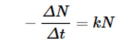Reaction Coordinate Diagrams

∙ a plot that shows energy (on the y-axis) as a function of the progress of the  reaction from reactants to products (on the x-axis)

o the conversion of reactants to products passes through a high-energy  state where an activated complex, or high-energy transition state, is  formed

o energy required to reach this transition state is the reaction’s

activation energy, EA.

 Activation energy is always a positive quantity and, for the

reaction where reactants are converted to products, is equal to

the energy difference between the reactant energy and the

energy of the activated complex

 Catalysts and temperature manipulation can change the

activation energy

∙ activation barrier

o an energy that must be overcome for reactants to proceed to form  products, and changing the temperature affects the ability of reactants to overcome this barrier

Arrhenius Equation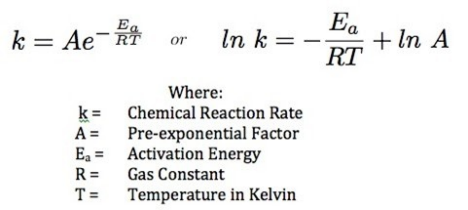∙ frequency factor, A, is a measure of the number of collisions that take place  with the correct orientation during a reaction

∙ frequency factor is a measure of the number of collisions that take place with  the correct orientation during a reaction

∙ as EA increases, the other e term decreases and the rate constant decreases;  and

∙ as T increases, the e term increases and the rate constant increases Graphical Determination of Activation Energy

∙ Arrhenius equation can also be used to graphically determine the activation  energy for a reaction using multiple temperature–rate constant data points  (second version above)

Components of Reaction Mechanism

∙ Reaction mechanism

o detailed description at the molecular level of steps by which reactants  are converted to products

o cannot be calculated or predicted with any certainty

o elementary step

 A simple event in which some chemical transformation occurs;  one of a sequence of events that form the reaction mechanism

 Gives overall equation for the reaction

 Molecularity of elementary step

∙ tells us how many species react in the step

∙ unimolecular

o involving a single reacting species

∙ bimolecular

o involving the collision of two reacting species

∙ termolecular

o three species collide simultaneously

o does not occur very often

o bond breaking step

 involves a single species breaking apart into two or more

species

o bond-forming step

 involves the combination of two or more species to form a single product

o rate-determining step

 rate of a reaction can be no greater than the rate of the slowest  step in the mechanism

∙ concerted process

o involves more than one chemical process happening simultaneously Multistep Mechanisms

∙ A chemical reaction that occurs in a single step has only one elementary  step, which is the same as the balanced equation for the chemical reaction ∙ Intermediates can be involved with multistep mechanisms

o species that do not appear in the overall net reaction

o species that is formed in one step in the mechanism and then  consumed in a later step

o A catalyst, a species that speeds up a chemical reaction, is consumed  in one step and then produced, in its original form, in a later step in the mechanism

o often short-lived chemical species that are very difficult to detect Reaction Mechanisms and the Rate Law

∙ the generalized rate law we wrote earlier for a chemical reaction, the reaction order (the exponents in the rate law) were unrelated to the reaction  stoichiometry

∙ the rate law for any elementary step in a mechanism is directly related to the stoichiometry of the elementary step because elementary steps describe the  molecular-level collisions that are occurring during the reaction

∙ rate law for each elementary step is equal to the rate constant for the  elementary step multiplied by the concentration of each reactant raised to  the power of its stoichiometric constant

∙ (dis)proving a mechanism

o Proposing a mechanism, including identifying the rate-determining  step.

o Predicting the rate law using the stoichiometry of the rate-determining  step.

o Determining the experimental rate law for the reaction using one of  the methods covered earlier in this unit. If the predicted and

experimental rate laws do not match, then the proposed mechanism is  incorrect. If they match, then the proposed mechanism might be  correct. However, the proposed mechanism might not be correct  because different mechanisms can lead to the same predicted rate law. Further experimentation is required to confirm whether the mechanism is correct or not.

∙ If both the forward and reverse reactions are fast and occur at the same rate,  a state of dynamic equilibrium is formed in which both reactants and  products are present in the reaction flask

∙ Advanced techniques for determining mechanisms

o Detection of an intermediate using spectroscopy

 Detection can be done using spectroscopic methods if the

intermediate absorbs light in the infrared, ultraviolet, or visible

region of the electromagnetic spectrum

o Trapping experiments

 experiments are used to chemically detect intermediates by  having the intermediate react with a secondary reagent to

produce an identifiable product

o Isotopic labelling

 one of the reactants is synthesized containing a particular

isotope of one of the reacting atoms

 location of the labeled atom can be tracked using a variety of  detection methods

Catalysis

∙ he technique of using a catalyst to influence the rate of a reaction. Catalysts  serve one of two functions: making reactions faster or making them more  “selective”

∙ the catalyst provides an alternative mechanistic pathway for the reaction,  which changes the activation energy and changes the reaction rate ∙ selectivity

o A measure of the tendency of a reaction to form one set of products  over another

o often a chemical reaction can proceed by many different pathways,  often with similar activation energies, which results in the formation of  many different products

∙ homogeneous

o often a chemical reaction can proceed by many different pathways,  often with similar activation energies, which results in the formation of  many different products

∙ heterogeneous

o not in the same phase as the compounds undergoing reaction

Page ExpiredIt looks like your free minutes have expired! Lucky for you we have all the content you need, just sign up here